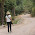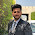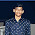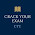## Sunday, 6 January 2013

### CBSE Class 9 - Chemistry - CH4 - Structure of Atom (MCQs)

Structure of AtomBohr Model credits:clker.com

MCQs

Q1: Proton was discovered by:

(a) Rutherford
(b) Goldstein
(d) J.J. Thomson

Q2: Canal rays are _______________

(a) negative charged particles
(b) positive charged particles
(c) beam of neutrons

Q3: α-particles are ___________

(a) negative charged particles
(b) positive charged particles
(c) beam of neutrons

Q4:  An α-particle is _____________

(a) a Hydrogen nucleus
(b) a Helium nucleus
(c) a proton
(d) an electron

Q5: Rutherford's gold foil experiment showed that most of the α-particles passed through the gold foil without any deflection. It indicates that

(a) the nucleus is concentrated at the centre
(b) the nucleus carries positive charge
(c) there is lot of empty space in atom
(d) the nucleus carries the most of the mass

Q6: Two elements X and Y have the same atomic mass but their atomic numbers are 20 and 21 respectively. X and Y are:

(a) isobars
(b) isotones
(c) isomers
(d) isotopes

Q7: In an atom, the mass number of an atom is equal to the number of _________________.

(a) nucleons
(b) protons
(c) electrons
(d) neutrons

Q8: Number of neutrons present in Hydrogen atom is _______.

(a) 0
(b) 1
(c) 2
(d) 3

Q9: Protium, Deutrium and Tritium are isotopes of ____________.

(a) Rhodium
(b) Sodium
(c) Hydrogen
(d) Helium

Q10: If Z represents the atomic number and A represents mass number, then the number of neutrons in an atom can be computed as

(a) A + Z
(b) A – Z
(c) Z – A
(d) Z

Q11: If Z represents the atomic number and A represents mass number, then the number of electrons  in an atom can be computed as

(a) A + Z
(b) A – Z
(c) Z – A
(d) Z

Q12: The electronic configuration of an atom is 2,8,3. The number of valence electrons in the atom is

(a) 13
(b) 10
(c)  3
(d)  8

Q13: The number of electrons in the outermost shell in the atom of an inert element is

(a) 0
(b) 1
(c) 2
(d) 8

Q14: Which one of the following will have the maximum charge/mass ratio?

(a) electron
(b) proton
(c) neutron
(d) α-particle

Q15: The maximum number of electrons that can be accommodated in M shell is:

(a) 2
(b) 8
(c) 18
(d) 32

1: (b) Goldstein
2: (b) positive charged particles
3: (a) negative charged particles
4: (b) a Helium nucleus
5: (c) there is lot of empty space in atom
6: (d) isotopes
7: (a) nucleons
8: (a) 0
9: (c) Hydrogen
10: (b) A – Z
11: (d) Z
12: (c)  3
13: (d) 8
14: (a) electron

15: (c) 181.P.S. Ans of 2 should be (a), 3 (b) and 6(a)...

2.And for 3 is doubly ( positively and negatively) charged

1.3.useful to children at basics level

4.useful to children at basics level

5.Answer of 1st ques. is Rutherford

6.is 7 ans is neutron or nucleons

1.7.is 7 ans is neutron or nucleons

8.It is nucleon because the mass number of an atom is the sum of the protonsame and neutrons present in the atom.They are counted as nucleoside because they are inside the nucleus

9.Please provide correct answers, otherwise close this

10.This comment has been removed by a blog administrator.

11.Answer for 6 question is isobars